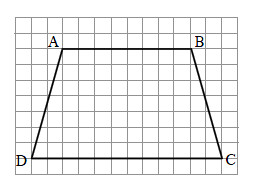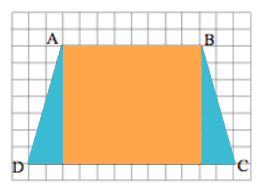### Home > MC2 > Chapter 2 > Lesson 2.3.4 > Problem2-131

2-131.

Copy the trapezoid below on grid paper.1. Find the length of the bottom base (segment $CD$), the length of the top base (segment $AB$), and the distance between the two bases. Use grid units.

Check that the length of $AB$ is $8$ units. Then find the length of $CD$ and the height.

2. Use what you learned about cutting up figures to find the area of the trapezoid.

One way to do this is to break up the trapezoid like this. Find the area of each part and then find the total area.